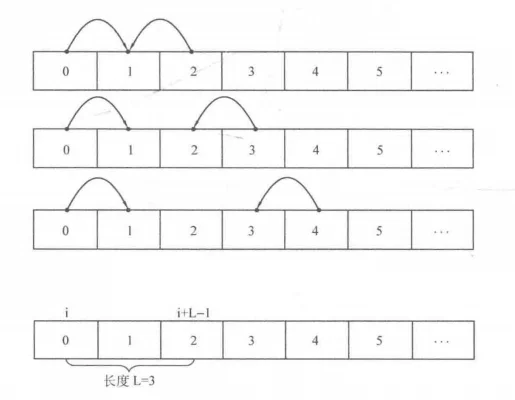0%

# leetcode 5 最长回文子串：

s[i]==s[[j],那么只要s[i+1][j-1]是回文子串，s[i]至s[j]就是回文子串；如果s[i+1][j-1]不是回文子串，则s[i]至s[j]也不是回文子串。
s[i]!=s[j]，那么s[i]至s[j]一定不是回文子串。

$dp[i][j] = \begin{cases} dp[i+1][j-1], & \text{s[i]==s[j]} \\ 0, & \text{s[i]!=s[j]} \end{cases}$1 <= N <= 1000

# leetcode 303 区域和检索

sumRange(0, 2) -> 1
sumRange(2, 5) -> -1
sumRange(0, 5) -> -3